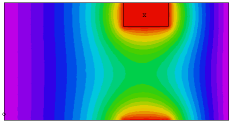﻿ Sample Problems > Usage > Periodicity > 3d_zperiodic

# 3d_zperiodic

Navigation:  Sample Problems > Usage > Periodicity >

# 3d_zperiodic{  3D_ZPERIODIC.PDE

This example shows the use of FlexPDE in 3D applications with periodic

boundaries in the Z-direction.

For Z-periodicity, we merely precede  the EXTRUSION statement by the

qualifier PERIODIC.  The top and bottom surfaces are assumed to match,

and values are made equal on the two surfaces.

In this problem we have a heat equation in an irregular figure.

An off-center source heats the body, while all the vertical surfaces are

held at U=0.

 }   title '3D Z-PERIODIC BOUNDARY TEST'   coordinates    cartesian3   Variables     u   definitions    k = 0.1    h=0    x0=0.3  y0=-0.2    x1=0.7  y1 = 0.2   equations    u : div(K*grad(u)) + h = 0   periodic extrusion z=0, 0.8, 1   boundaries   Region 1     start(-1,-1)       value(u)=0     line to (1,-1)     arc(center=-1,0) to (1,1)     line to (-1,1)     arc(center=-3,0) to close{ an off-center heat source in layer 2 provides the asymmetric

conditions to demonstrate the periodicity of the solution }

limited region 2

layer 2 h=10 k=10

surface 1 { include insert patch in surface 1 so surfaces match }

start(x0,y0) line to (x1,y0) to (x1,y1) to (x0,y1) to close

monitors

contour(u) on y=0

plots

grid(x,z) on y=0

contour(u) on y=0 painted

end